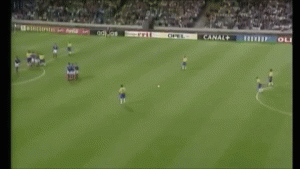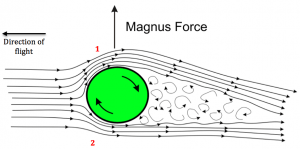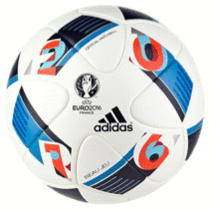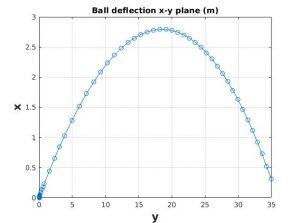# Football free-kicks… taken by Newton

Discover the mysteries behind the most spectacular free-kick ever scored and how Newton can help us to simulate it.This Saturday might be a normal day for many people, but football fans around the world are looking forward to it, as two of the best teams in the the world are playing in the final of the best club football competition, the Champions League, at the National Stadium of Wales in Cardiff.

Here at Chalkdust, we’re quite excited too, and so we decided to analyse the mathematics and physics behind one of the best goals ever scored… and go on to reproduce it mathematically!  You might find it provides an excellent topic of conversation in your preferred pub before the match or during half-time.

## The impossible football free-kick

#### The game

In 1997, the Tournoi de France (French for “Tournament of France”) was an international football tournament organised by France, held the year before the World Cup was hosted by the same country. Four national teams participated: France, Italy, England and Brazil. The teams played against each other and England, having won the most points, finished as the champions. This might sound like any other normal tournament, but something happened that no one had seen before.

On 3rd June, the inaugural match took place in Lyon: France played against the reigning World Cup champions Brazil—a match that every football fan would have loved to watch, as many of the most gifted and decorated players of their generation (even of all time) were playing. These included Zinedine Zidane (one of the best French players in history), Fabian Barthez, Ronaldo Nazario (the best and most skilful striker at the time), Romario, Cafu and Roberto Carlos.

#### The goal

One of the French players committed a foul towards the middle of the field, 35 metres away from the goal France was defending. The Brazilian left-back, Roberto Carlos, who was known to be an excellent free-kick taker, took the ball.  Although it was a long range free-kick, the goalkeeper Fabian Barthez knew all about Roberto Carlos’ ability (his powerful free-kicks had been measured at 169km/h), and so he placed four players in the wall to block the shot (normally, from long distance, a goalkeeper would place only two players). Roberto Carlos took a long run-up in order to hit his shot with lots of power and then… he made it.  It was astonishing! Take a look for yourself:Impressive, isn’t it? What a goal. So powerful. But now, let’s change the angle of the camera:I would use many adjectives to describe this goal, but the space I have is simply too short to write them all down. Simply incredible. Many (older) football fans will recognise this goal, but if this is the first time you watch it, I hope it gives you the same emotions it gave me when I watched this beauty at 11 years of age. This is by far the most spectacular goal I have seen so far in my life: from 35m out the ball was heading towards the corner flag, but it then curved like a “banana” and landed in the back of the net. What made it even more impressive is that the bend was so pronounced the French goalkeeper never even moved. You can actually see that in the GIF above. Even the camera man was tricked by Roberto Carlos’ shot.

This free-kick caught the attention of many scientists, whose main concern was to explain the banana effect of the ball’s trajectory and under what conditions the famous free-kick could be reproduced.  Match commentators at the time named it the impossible free kick, or the goal that defied the laws of physics. We will see that in fact there was nothing impossible about it at all and that it actually perfectly obeyed the laws of physics.

## The Magnus effect

The phenomenon responsible for the banana effect is actually very common in ball sports. In fact, here at Chalkdust we have already written about its appearance in the world of tennis. The phenomenon is known as the Magnus effect, which can be explained using a simple illustration.Diagram that shows a rotating ball moving through air. By Rdurkacz – using inkscape, inspired by original version of the Wikipedia diagram, CC BY-SA 3.0,

In the diagram, the football is moving to the left and is also rotating clockwise at the same time. When this happens, there is a thin layer of fluid (in our case, air) that moves with the surface of the ball. This causes a difference in the velocities of the air at the upper (1) and lower (2) sides of the ball.At position 1, the football is rotating away from the direction of the ball’s flight. If we measured the speed of the air flow at that point, we would observe that the velocity would always be smaller than the velocity on the bottom side. This is because at position 2, the ball is moving faster due to the combined effect of the flight direction and rotation. The difference in velocities on the two sides of the ball will cause a pressure difference resulting in a net force acting perpendicularly to the direction of flight. This is known as the Magnus force.

#### Roberto Carlos’ physique

We can now give a simple explanation of Roberto Carlos’ free-kick: he struck the ball with the outer side of his boot’s laces, which caused the ball to move through the air with a large amount of rotation. This generated the Magnus force that pushed the ball to the left and made it enter the goal.

However, a spinning ball is not enough: the ball has to be hit strongly enough to induce enough spin, which is something that Roberto Carlos knew how to do perfectly.  If a free-kick like Roberto Carlos’ is to be replicated, it needs to be from long range to ensure that the Magnus force has enough of an effect on the ball before it reaches the goalpost. If the distance from the free-kick taker and the goal are not large enough, the football would just end up going well wide of the goal.

## Newton’s second law

#### Forces on a ball

Now that we know the science behind Roberto Carlos’ free kick, it is time to start using mathematics to model the ball’s trajectory. First of all, we need to determine the forces acting on the ball. These are illustrated in the diagram:

The ball (with mass $m$) is moving through the air (of density $\rho$) with vector velocity $\vec{v}=(v_x, v_y, v_z)$. The simplest force acting on the ball is the gravitational force ($m\vec{g}$), which is pointing downwards and is assumed to be constant.

There are two additional forces that only exist when the ball is moving through a fluid: one of them is called the drag force ($\vec{F}_D$) and it is related to the air resistance. The main characteristic of this force is that it acts in the opposite direction to the motion of the ball. Mathematically speaking, the drag force adopts the following form:

$\vec{F}_D = -\frac{1}{2}\rho A C_D|\vec{v}|\vec{v},$

where $A$ is the cross-sectional area of the ball; $|\vec{v}|$ is the magnitude of the vector velocity; and $C_D$, a dimensionless number, is the drag coefficient, which has to be determined experimentally and depends on many factors, such as the characteristics of the ball and the temperature, among others. Greater values of $C_D$ mean higher air resistance, and in the absence of air, $\vec{F}_D=0$.

The other force is the Magnus force, $\vec{F}_L$ (also called the lift force). As we stated, this force is the result of the ball interacting with air: the main difference with the drag force is that the spin of the football plays an important role here.  The lift force is perpendicular to the vector velocity, and can be calculated using the following relation:

$\vec{F}_L = \frac{1}{2}\rho A C_L|\vec{v}| f(\theta)\vec{v}.$

As we can see, the Magnus force depends on almost the same variables as the drag force, except that instead of a drag coefficient, now we have a lift coefficient $C_L$ (which also has to be calculated experimentally) and there is a function $f(\theta)$ that relates the Magnus force with the angle of spin of the ball.

#### Newton’s second law has a ball!

As we all know, Newton’s second law simply states that the rate of change of momentum ($m\vec{a}$, when $m$ is constant) is equal to the forces acting on a body (in our case, the ball). Summing all the forces, we end up with the general equation we are looking for:

$m\vec{a} = -m\vec{g} + \vec{F}_D + \vec{F}_L.$

All the terms of the equation are in vector form. We wish to obtain and describe the trajectory of a spinning ball in three dimensions, $\vec{x}=(x,y,z)$. Assuming  that the angle of spin is $-90$º, the simplest system of equations to trace the football’s trajectory is:

$$\frac{\mathrm{d}^2x}{\mathrm{d}t^2} = -|\vec{v}|H \Big(C_D\frac{\mathrm{d}x}{\mathrm{d}t} + C_L \frac{\mathrm{d}y}{\mathrm{d}t}\Big)$$

$$\frac{\mathrm{d}^2 y}{\mathrm{d}t^2} = -|\vec{v}|H \Big(C_D\frac{\mathrm{d}y}{\mathrm{d}t} – C_L \frac{\mathrm{d}x}{\mathrm{d}t}\Big)$$

$$\frac{\mathrm{d}^2 z}{\mathrm{d}t^2} = -|\vec{v}|H C_D\frac{\mathrm{d}z}{\mathrm{d}t}- g,$$

where $H$ and $|\vec{v}|$ are:

$$H= \frac{\rho A}{2m}$$

$|\vec{v}|= \sqrt{ (\frac{\mathrm{d}x}{\mathrm{d}t})^2 + (\frac{\mathrm{d}y}{\mathrm{d}t})^2 + (\frac{\mathrm{d}z}{\mathrm{d}t})^2}$.

We have a system of three ordinary differential equations (ODEs) in three dimensions whose dependent and independent variables are position and time, respectively. Notice that the drag forces ($C_D$) will affect the motion in all three dimensions. On the other hand, the Magnus force ($C_L$) will only have an effect on the $x$-$y$ plane because these equations consider that the ball is spinning about its vertical axis ($z$). The equations also assume that the gravitational force will just affect the motion in $z$.  Now that we have the necessary equations, it is time to solve them.

## Simulating Roberto Carlos’ free-kick

In order to completely solve the system of ODEs, we need the initial conditions ($\vec{x}_0$, $\vec{v}_0$), empirical equations to calculate the coefficients $C_D$ and $C_L$, the characteristics of the ball ($m$ and $A$) and the density of air ($\rho$).

#### Drag and lift coefficients

The drag and lift coefficients need to be determined experimentally: in a laboratory, the trajectory of a football is studied and its velocity and acceleration are then calculated. Once this information is known, equations for $C_D$ and $C_L$ as a function of the speed and other parameters are derived. Many equations for these coefficients can be found in the literature. Here we will use the simplest ones: for instance, many authors have proposed an equation for the lift coefficient that adopts the following form:

$$C_L = \frac{\omega R}{|\vec{v}|},$$

where $R$ and $|\vec{v}|$ are the ball’s radius and velocity, respectively, and $\omega$ is the angular velocity of the ball, which is dependent on time:

$$\omega = \omega_0 \exp{(-t/7)}.$$

Here $w_0$ is the angular velocity that the ball has when it is first hit by the player. We can notice that the angular velocity decreases as time increases; and if the lift coefficient is proportional to the angular velocity, then $C_L$ will also decrease, and therefore we can say that the Magnus force will affect the trajectory of the ball at short to medium times, and at large times its effect will vanish. It has been reported that the initial angular velocity of Roberto Carlos’ free kick was 88 rad/s, so we will use this value later.

The equations for estimating the drag coefficient are more complex and more complicated to obtain experimentally, as $C_D$ depends on many factors. For instance, in this paper they determined the following equation for a Teamgeist ball (used in the World Cup of Germany, 2006):

$$C_D= \begin{cases} 0.4127(C_L)^{0.3056} &\mbox{if } |\vec{v}|> v_c &\mbox{and} \qquad C_L >0.05 , \\ 0.155 + \frac{0.346}{1+ \exp{(|\vec{v}|- v_c)/v_s}} &\mbox{otherwise,} \end{cases}$$

where $v_c=12.19$m/s and $v_s=1.309$m/s.  The sudden change in the values of the drag coefficient is due to a phenomenon called drag crisis, which is commonly observed in fluid mechanics problems.

#### Characteristics of the ball

We will be using the following values for the radius $R$ and mass $m$ of a standard football, and the density of the air (at standard temperature and assuming that it is a constant):

$m=0.442 \mbox{kg} \qquad R=0.11 \mbox{m} \qquad \rho = 1.225 \mbox{kg}/\mbox{m}^3$

#### Initial conditions

We have a system of three ordinary differential equations of second order, which means we need six initial conditions in order to find the solution for the system: three of them are for the initial vector position $\vec{x}_0$ and the other three for the initial vector velocity $\vec{v}_0$.

For practical purposes, using a Cartesian coordinate system, we choose the zero vector $\vec{x}_0=(0,0,0)$ to be the point from which the free kick is going to be taken. The initial vector position, on the other hand, is not as simple to obtain. We will make use of the following figure, which illustrates our coordinate system:

The ball will move with an initial vector velocity $\vec{v}_0=(v_{x0}, v_{y0},v_{z0})$, whose magnitude is defined as:

$|\vec{v}_0|= \sqrt{ (v_{x0})^2 + (v_{y0})^2 + (v_{z0})^2}$.

It has been reported that the initial velocity of the ball in Roberto Carlos’ free-kick was approximately $38$m/s. The values of the angle with the horizontal, $\alpha$, and the angle to the $y$-axis, $\gamma$, were also determined by scientists and their values are

$\alpha = 16º \qquad \gamma=5º.$

These values are highly important as they will allow us to calculate the elements of the initial vector velocity. Using a bit of geometry and trigonometry, and taking the values of the angles $\alpha$, $\gamma$ and $|\vec{v}_0|=$38 m/s, we can calculate the values of the components of the initial vector velocity, which are:

$$\vec{v}_0=(3.18, 36.39, 10.47)\mbox{m/s}$$

Now we have all the elements necessary to solve our system of ODEs. However, an analytic solution is not possible to obtain, as there are non-linear terms and the system of equations is strongly coupled: thus, numerical methods are required. We will use one of the most popular software packages, MATLAB, to find a numerical solution to our system. More specifically, we will use the solver called ode45, which, for a given set of initial conditions, will help us to integrate our equations.

#### Simulation results

Once we have introduced our system of equations, the parameters (lift and drag coefficients, characteristics of the ball) and the initial conditions, we make use of the solver ode45 and we then plot the solutions in our coordinate system. In our first calculation we consider the initial angular velocity as zero ($\omega_0=0$). Our code gives us the following results:

The red lines represent the dimensions of the goal according to official rules of football (just half of it is shown as the free kick is taken from a position roughly at the centre of the goal). For this case, notice that the trajectory of the ball, which adopts a nice parabolic form, is not even close to the goal, which is 35m away along the $y$-axis from the initial point $\vec{x}_0=(0,0,0)$. Now we show the results considering the exact conditions of Roberto Carlos’ free-kick, setting the angular velocity to be 88 rad/s.

Goal!  (Kind of!)  The banana effect described at the beginning of this blog post can be replicated using a simple model that has been derived from Newton’s second law. According to our calculations, it took approximately 1.34s for the ball to reach the goal, which is consistent with the actual free kick. Below, we can appreciate the ball deflection in the $x$-$y$ plane:Assuming that the the wall formed by four players is located 9.1m from the point where the free kick is taken (according to the official rules of football) and has a width of 1m width, our predictions show the ball sidestepping the wall and beginning to bend inwards approximately 20m away from our point of origin. It has been reported that the deflection in Roberto Carlos’ free kick was approximately 3m, which is also consistent with these results.

However, for the ball height, things are slightly different. In the diagram below, we show the ball height in the $y$-$z$ plane:The figure shows that the ball reaches a maximum height of approximately 4m, but that its final height (when the ball has travelled 35m in the $y$-direction) is around 2.5m, which is 1 metre greater than the final height seen in the real free-kick and higher than the actual crossbar! The difference is mostly due to the equations for the drag coefficient $C_D$, which have been calculated for a Teamgeist ball, a football with different characteristics than the one used in the match played in 1997. In addition, we are using a simple model derived from Newton’s second law, and we are ignoring a possible contribution of the Magnus force in the equation for the acceleration in $z$ direction.

Although there are many more sophisticated and complex models (some of them using the approach of fluid mechanics), the model derived from classical mechanics can replicate nicely the trajectory of the ball and the banana effect observed in the famous Roberto Carlos free-kick.As mathematicians and scientists, it would be great to think that Roberto Carlos spent weeks solving differential equations and studying the physics behind the ball’s motion.  But probably not. In reality, professional players spend months and years training, and they manage to learn and understand through pratice phenomena such as the Magnus force and how air resistance can affect their shots: the result is that we have witnessed some spectacular goals.

For further information on this topic, I highly recommend the article Football curves, written by Gillaume Dupeux and colleagues, in which they analysed all the curves observed in football. In 2010, they carried out a more in-depth analysis into the problem and simulated the free kick in question perfectly. In addition, they demonstrated that if gravity had not taken over, the path the ball would have taken would have adopted the form of an ideal spiral.

Now that you know a bit about the physics and mathematics behind free-kicks, it’s time to go to the pub and support your favourite team and, maybe, see the Magnus effect in action!

#### References

1. Sandhu, J., Edgington, A., Grant, M., Rowe-Gurney, N., How to score a goal, Journal of Physics Special Topics, 2011.
2. Goff, J.E., Carré, M., Soccer ball lift coefficients via trajectory analysis, European Journal of Physics, May 2010, Vol 31, No 4.
3. Goff, J.E., Carré, Trajectory analysis of a soccer ball, American journal of Physics, 77, 1020 (2009).
4. Bray, K., Kerwin, D., Modelling the flight of a soccer ball in a direct free kick, Journal of Sports Science, Vol. 21, Issue 2, 2003.
5. Dupeux, G., Cohen C., Le Goff., A., Quére, D., Clanet, C., Football curves, Journal of Fluids and Structures, Vol. 27, Issues 5-6, 2011.
6. Hazar, Alexandra, Arsic, D., Diaz, J.J., Hakansson, J., Carvalho, P.F., Paas, R., Reis, T., Phantom footballs and impossible free kicks: modelling the flight of modern soccer balls, Modelling week, final report, TUD, ECMI, 2012.